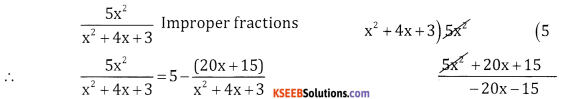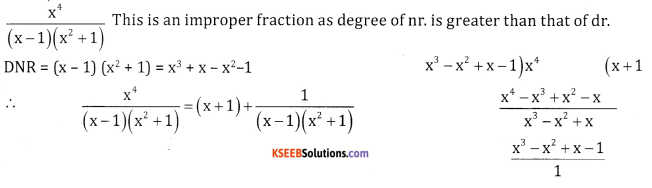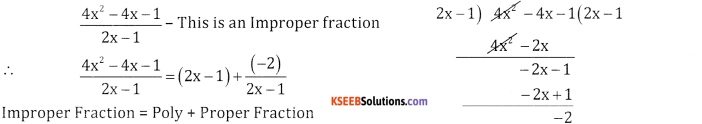# 2nd PUC Basic Maths Question Bank Chapter 5 Partial Fractions Ex 5.1

Students can Download Basic Maths Exercise 5.1 Questions and Answers, Notes Pdf, 2nd PUC Basic Maths Question Bank with Answers helps you to revise the complete Karnataka State Board Syllabus and score more marks in your examinations.

## Karnataka 2nd PUC Basic Maths Question Bank Chapter 5 Partial Fractions Ex 5.1

Part – A

2nd PUC Basic Maths Partial Fractions Ex 5.1 Two or Three Marks Questions and Answers

Question 1.
Express the following as a sum of polunomial and proper rational fraction ; $$\frac{x^{2}+x+1}{x^{2}-1}$$Question 2.
$$\frac{3 x^{2}-4 x+7}{x+7}$$Question 3.
$$\frac{x^{2}-1}{x^{2}+1}$$Question 4.
$$\frac{5 x^{2}}{x^{2}+4 x+3}$$Question 5.
$$\frac{x^{4}}{(x-1)\left(x^{2}+1\right)}$$Question 6.
$$\frac{4 x^{3}-2 x^{2}+3 x+1}{2 x^{2}+4 x-1}$$Question 7.
$$\frac{4 x^{2}-4 x-1}{2 x-1}$$$$\frac{x^{3}+7}{x^{2}-2 x+1}$$